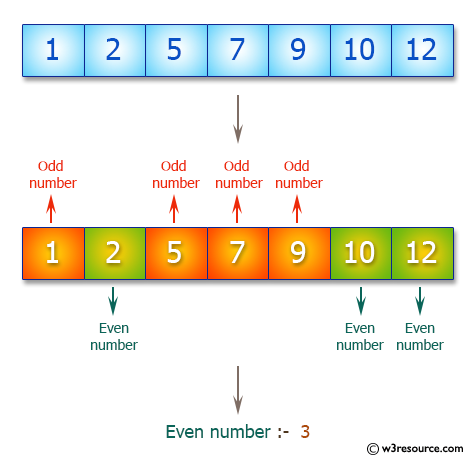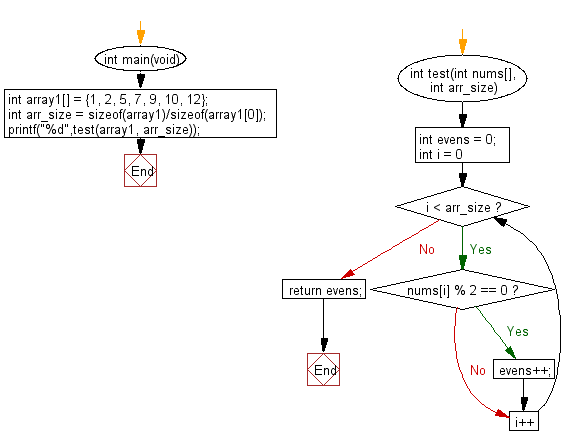﻿ C Program: Count even elements in an array of integers# C Exercises: Count number of even elements in a given array of integers

## C-programming basic algorithm: Exercise-51 with Solution

Write a C program to count number of even elements in a given array of integers.

C Code:

``````#include <stdio.h>
#include <stdlib.h>
int main(void){
int array1[] = {1, 2, 5, 7, 9, 10, 12};
int arr_size = sizeof(array1)/sizeof(array1);
printf("%d",test(array1, arr_size));
}
int test(int nums[], int arr_size)
{
int evens = 0;
for (int i = 0; i < arr_size; i++)
{
if (nums[i] % 2 == 0) evens++;
}
return evens;
}
``````

Sample Output:

```3
```

Pictorial Presentation:Flowchart:C Programming Code Editor:

What is the difficulty level of this exercise?

Test your Programming skills with w3resource's quiz.

﻿

## C Programming: Tips of the Day

Why doesn't a+++++b work?

printf("%d",a+++++b); is interpreted as (a++)++ + b according to the Maximal Munch Rule!.

++ (postfix) doesn't evaluate to an lvalue but it requires its operand to be an lvalue.

! 6.4/4 says the next preprocessing token is the longest sequence of characters that could constitute a preprocessing token"

Ref : https://bit.ly/3fdldUT